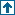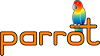parrotcode: Some utility functions Contents | C

# NAMEsrc/utils.c - Some utility functions

# DESCRIPTIONPrototypes are in src/misc.h.

Opcode helper functions that don't really fit elsewhere.

## Functions`INTVAL intval_mod(INTVAL i2, INTVAL i3)`

NOTE: This "corrected mod" algorithm is based on the C code on page 70 of . Assuming correct behavior of the built-in mod operator (%) with positive arguments, this algorithm implements a mathematically convenient version of mod, defined thus:

`    x mod y = x - y * floor(x / y)`
For more information on this definition of mod, see section 3.4 of , pages 81-85.

References:

 Donald E. Knuth, *MMIXware: A RISC Computer for the Third Millennium* Springer, 1999.

 Ronald L. Graham, Donald E. Knuth and Oren Patashnik, *Concrete Mathematics*, Second Edition. Addison-Wesley, 1994.

`FLOATVAL floatval_mod(FLOATVAL n2, FLOATVAL n3)`

Returns `n2 mod n3`.

Includes a workaround for buggy code generation in the `lcc` compiler.

## Random Number GeneratorBased on the `rand48()` family of functions.

`static void next_rand(_rand_buf X)`

Returns the next random number in `X`.

`static FLOATVAL _erand48(_rand_buf buf)`

Returns a `double` in the interval `[0.0, 1.0)`.

`static FLOATVAL _drand48(void)`

Returns a `double` in the interval `[0.0, 1.0)`.

`static long _jrand48(_rand_buf buf)`

Returns a `long` in the interval `[-2^31, 2^31)`.

`static long _nrand48(_rand_buf buf)`

Returns a `long` in the interval `[0, 2^31)`.

`static long _lrand48(void)`

Returns a `long` in the interval `[0, 2^31)`.

`static long _mrand48(void)`

Returns a `long` in the interval `[-2^31, 2^31)`.

`static void _srand48(long seed)`

Sets the high order 32 bits to the argument `seed`. The low order 16 bits are set to the arbitrary value 0x330e.

`FLOATVAL Parrot_float_rand(INTVAL how_random)`

Returns a `FLOATVAL` in the interval `[0.0, 1.0)`.

`how_random` is ignored.

`INTVAL Parrot_uint_rand(INTVAL how_random)`

Returns an `INTVAL` in the interval `[0, 2^31)`.

`how_random` is ignored.

`INTVAL Parrot_int_rand(INTVAL how_random)`

Returns an `INTVAL` in the interval `[-2^31, 2^31)`.

`how_random` is ignored.

`INTVAL Parrot_range_rand(INTVAL from, INTVAL to, INTVAL how_random)`

Returns an `INTVAL` in the range `[from, to]`.

`how_random` is ignored.

`void Parrot_srand(INTVAL seed)`

Seeds the random number generator with `seed`.

## Array Functions`void *Parrot_make_la(Interp *interpreter, PMC *array)`

Creates a C array of `long`s with one more element than the number of elements in `*array`. The elements are then copied from `*array` to the new array, and the last (extra) element is set to 0.

Used in `src/nci.c`.

`void Parrot_destroy_la(long *array)`

Use this to destroy an array created with `Parrot_make_la()`.

`void *Parrot_make_cpa(Interp *interpreter, PMC *array)`

Creates a C array of `char *`s with one more element than the number of elements in `*array`. The elements are then copied from `*array` to the new array, and the last (extra) element is set to 0.

Currently unused.

`void Parrot_destroy_cpa(char **array)`

Use this to destroy an array created with `Parrot_make_cpa()`.

`PMC *tm_to_array(Parrot_Interp, struct tm *)`

Helper to convert a struct tm * to an Array

`typedef int (*reg_move_func)(Interp*, unsigned char d, unsigned char s, void *);`

`void Parrot_register_move(Interp *, int n_regs, unsigned char *dest_regs, unsigned char *src_regs, unsigned char temp_reg, reg_move_func mov, reg_move_func mov_alt, void *info)`

Move `n_regs` from the given register list `src_regs` to `dest_regs`.

```  n_regs    ... amount of registers to move
dest_regs ... list of register numbers 0..255
src_regs  ... list of register numbers 0..255
temp_reg  ... a register number not in one of these lists
mov       ... a register move function to be called to move one register
mov_alt   ... a register move function to be called to move one register
which triese fetching from an alternate src (or NULLfunc):

(void)  (mov)(interp, dest, src, info);
moved = (mov_alt)(interp, dest, src, info);```
Some `dest_regs` might be the same as `src_regs`, which makes this a bit non-trivial, because if the destination is already clobbered, using it later as source doesn"t work. E.g.

```  0 <- 1
1 <- 0     # register 0 already clobbered```
or

```  2 <- 0
0 <- 1
3 <- 2      # register 2 already clobbered - reorder moves```
To handle such cases, we do:

```  a) rearrange the order of moves (not possible in the first case)
and/or if that failed:
b) if an alternate move function is available, it may fetch the
source from a different (non-clobbered) location - call it.
if the function returns 0 also use c)
c) if no alternate move function is available, use the temp reg   ```
The amount of register moves should of course be minimal.

TODO add a define, if it's implemented so that we can start filling the gaps

# HISTORYInitial version by leo 2003.09.09.